6.4.4 Symmetric Case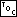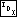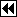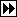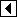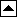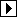```206. <Right-side rebalancing after RB insertion 206> =
struct rb_node *y = pa[k - 2]->rb_link;
if (y != NULL && y->rb_color == RB_RED)
{     <Case 1 in right-side RB insertion rebalancing 207>   }
else   {
struct rb_node *x;

if (da[k - 1] == 1)
y = pa[k - 1];
else       {         <Case 3 in right-side RB insertion rebalancing 209>       }

<Case 2 in right-side RB insertion rebalancing 208>
break;
}
```

This code is included in 201.

```207. <Case 1 in right-side RB insertion rebalancing 207> =
<Case 1 in left-side RB insertion rebalancing 203>
```

This code is included in 206, 346, and 463.

```208. <Case 2 in right-side RB insertion rebalancing 208> =
x = pa[k - 2];
x->rb_color = RB_RED;
y->rb_color = RB_BLACK;

pa[k - 3]->rb_link[da[k - 3]] = y;
```

This code is included in 206, 347, and 465.

```209. <Case 3 in right-side RB insertion rebalancing 209> =
x = pa[k - 1];